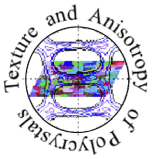EBSD and BKD

Pattern Solving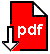1. Indexing a Kikuchi Pattern

A Kikuchi pattern can be interpreted as a gnomonic projection of the crystal lattice under the beam spot on the flat registration screen. The angles between the centerlines of bands correspond to interplanar angles of the crystal lattice, and the bandwidths correspond to the reciprocal interplanar spacings. This means that the geometry of a Kikuchi pattern is specific to the structure and the orientation of the crystallite. The most important step in pattern solving is to precisely determine the positions of Kikuchi Bands.

To index a Kikuchi pattern, one only needs to know the positions and widths of some bands. While a skilled user can easily detect even diffuse bands on a strong background, automatic band localization is a task not so easily accomplished with software. It has been proven in practice to locate the bands by applying a Radon transformation to the Kikuchi pattern.

The Radon transformation  is often defined in the 2-dim space R2 as a continuous function that projects the intensity distribution f(x, y) along a line S in an image into the 2-dim Radon space. The transformation process is defined by the line integral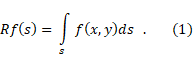In particular, a straight line S can be parametrized as bx + ay = ab (x-intercept y-intercept equation) or as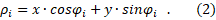Thus, the line integral can also be written as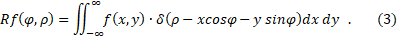ρ is the distance of the straight line from the coordinate origin and φ is the angle between the x-axis and the perpendicular to the origin (i.e. the point at the center). φ is limited to 0 ≤ 2π. δ denotes the Dirac delta function. Thus, a straight line in a 2D image domain is associated to exactly one point (ρ, φ) in the 2D Radon domain.

A discrete image, here the digitally recorded Kikuchi pattern, consists of a matrix f[x, y] of gray-scale image points called pixels. We must therefore consider a discrete Radon transformation, with the integrals in equations 1 and 3 replaced by sums. The image domain and the Radon domain consist of arrays of discrete cells on a Cartesian grid (x, y) and a grid (ρ , φ), respectively. The sizes of the cells have to be adjusted to the resolution of EBSD measurement. A Kikuchi band can be thought of as a bundle of straight lines slightly rotated and shifted with respect to each other. Thus, using the Radon transform in equation 3, the intensity profile of a band is mapped pixel by pixel not in a sharp point, as would be the case with a single straight line, but smeared out in a kind of "butterfly" motif in the Radon domain.

The concept of Radon transformation has some advantages for band localization [2, 3]. A clever approach is to traverse the domain in Radon space inversely cell by cell (ρ , φ), instead of scanning through the Kikuchi pattern directly and transforming pixel by pixel into the Radon domain. Thus, one first sums up the intensity values along the corresponding straight line, that is the projection line S, in the pattern and then writes, in one step, this value to the respective cell. Since successive points on the straight line are considered, the intensity profile along the line can be interrogated. For example, sections of the straight line have similar intensity inside a band and a cusp or a peak at the bordering Kikuchi lines. Short sections indicate lines that run (partly) outside a band, and these sections can be skipped during the transformation by applying a run-length filter. Similarly, artifacts and intensity peaks ("hot spots") can be masked out by applying local bounds along the intensity profile. Locally excessive intensity values occur at the zone axes, for example, while diffraction points or dynamic diffraction effects can cause unusually high or low intensity modulations. Applying a nonlinear intensity operator to the domain  can further suppress noise and effectively improve contrast. Overall, intensity evaluation along straight lines with the "inverse" construction of the Radon transform results in a clean domain in Radon space.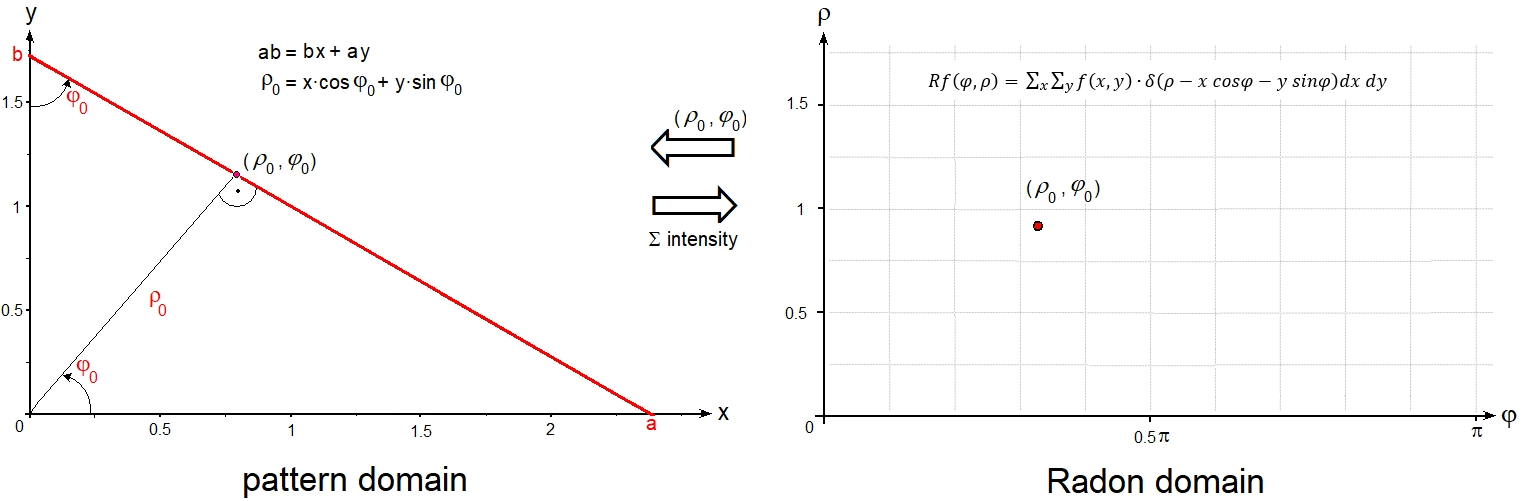An image similar to the pattern can be reconstructed from its Radon transform using the back-projection operator: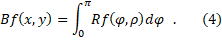The inverse transform specifies which straight line in the image space is associated with each cell in the Radon domain, and the stored intensity is equally attributed to all points on that line. The Radon backprojection does not provide the original image, but produces a smoothed image. In general, statistical noise is reduced. The Kikuchi bands in a pattern are bordered by slightly hyperbolic instead of straight lines.This may cause a further blur in back projection.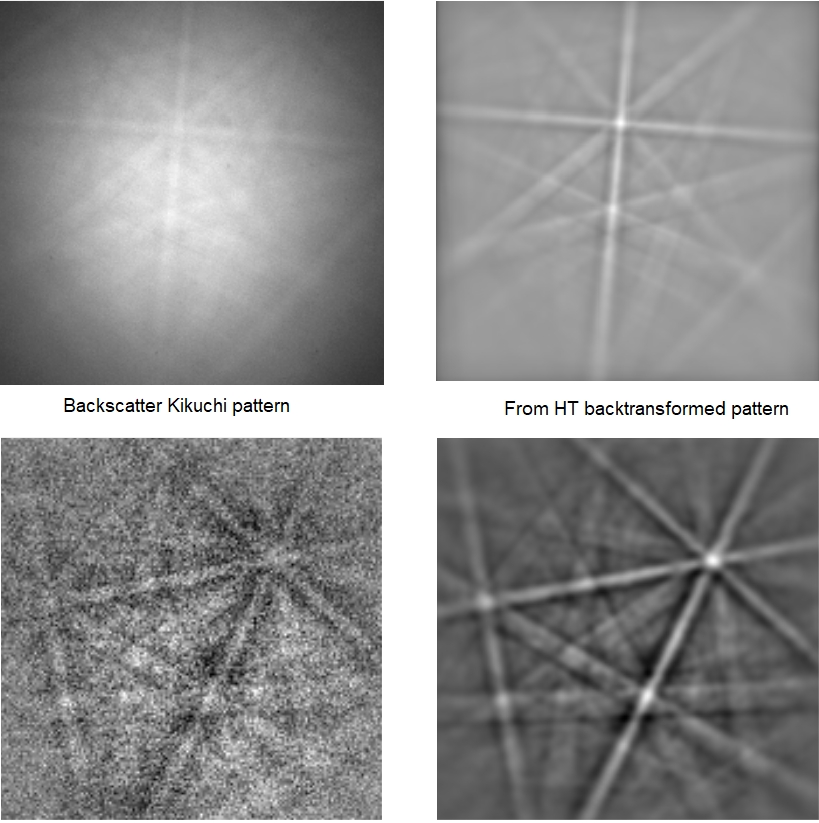Applying a Radon transformation to the Kikuchi pattern simplifies the inherently difficult task of locating lines and bands, since only isolated peaks in the Radon domain need to be found. The "butterfly-like" peaks are best located by a peak search with constraints or by evaluating some coefficients of a 1D FFT of the Radon domain along ρ-direction. These peaks are assigned to Kikuchi bands in the pattern according to the inverse Radon transform .

2b. The Hough Transformation

Forty-five years after the publication of the Radon transformation, the Hough transformation was proposed by Paul V.C. Hough in a patent . The underlying objective was to locate straight lines in a discrete image with binary intensity distribution fb(x, y), which originally represented particle tracks in a bubble chamber. With a more convenient slope-intercept parametrization of y = b - (b/a)·x is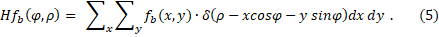The image domain and the Hough domain are 2-dimensional spaces with Cartesian coordinates (x, y) and (ρ , φ), respectively. The common interpretation is that each point (x, y) in the image space is transformed into a sinoidal curve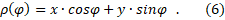If points (x, y) in the image lie on a straight line, their sinoidals intersect at a common point and their intensity is accumulated to a peak in the Hough domain.In a binary image, pixels only take a value of either 1 or 0, so the value on each sinoidal is either 1 or 0. The Hough transform is a particularly fast method to locate sparse straight lines with isolated intensity values = 1, because pixels with intensity values = 0 are skipped during the transformation. Only for pixels with fb(x, y) = 1 the sinoidals are calculated and get intensity = 1. Thus, the height of the Hough peaks is equal to the number of intersecting sinoidals, i.e. the number of non-zero points on the corresponding line in the image. The discretization, inverse transform, and subsequent detection of bands are the same as described above for the Radon transform.

Originally, the discrete Hough transform was defined only for binary (bitonal, 1-pixel) images. In a first step, grayscale images are converted to binary images by an edge filter before the Hough transform is carried out. Since Kikuchi patterns are gray-scale and not binary images, a Hough transform is, strictly speaking, not well suited for band detection. Of course, a gray-scale Kikuchi pattern can be reduced to a binary pattern, and then Hough transformed. The result is a significant loss of information, especially with respect to the band profile used to evaluate a meaningful Pattern Quality (PQ). On the other hand, the speed is increased by about a factor of 1/3, depending on the threshold of binarization (0 or 1) of the intensity levels. Another disadvantage of applying a Hough transform to binary Kikuchi patterns is a lower accuracy of orientation data. A better approach to increase speed, at the expense of accuracy, is to work with a higher binning rate, e.g. 60x60 pixels instead of 100x100 or more pixels per pattern.

The discrete Hough transform was therefore "modified" in  to account for and evaluate gray-scale patterns. Thus it loses the advantage of high speed by skipping pixels with zero intensity. The result is quite similar to that of the Radon transformation without the run-length filter as discussed above. The theory and implementations of Radon and Hough transformations are presented in detail in [6, 7]. The Hough transformation and, moreover, its modification for grayscales are special cases of the Radon transformation. The essential difference between the Radon transform and the "modified" Hough transform in BKP analysis lies in the method of how the transformed domains are constructed from the image domain.

In early EBSD analyses, peaks were located in the "Hough" domain by applying a normalized cross-correlation process with a large "butterfly-shaped" filter mask. In the simplest model, a rectangular pot profile was applied to the band intensity . The main drawbacks of this method are the dependence of the band profile on the position of the band in the Kikuchi pattern, the type of pattern, the diffraction geometry, the accelerating voltage, the grating type, and the {h k l} of the band to be detected. To satisfy this, the filter mask must be matched to the current band profile. Incorrectly matched masks will result in inaccurately determined band positions and bandwidths. The wider a band is, the larger the mask would have to be. Large masks increase the computing time extremely. A single mask is generally not sufficient; several masks are required to analyze a pattern. Overall, cross-correlation with a filter mask tends to be ineffective.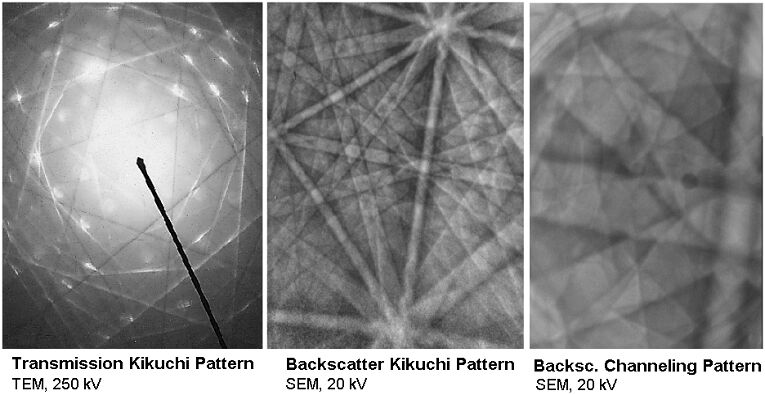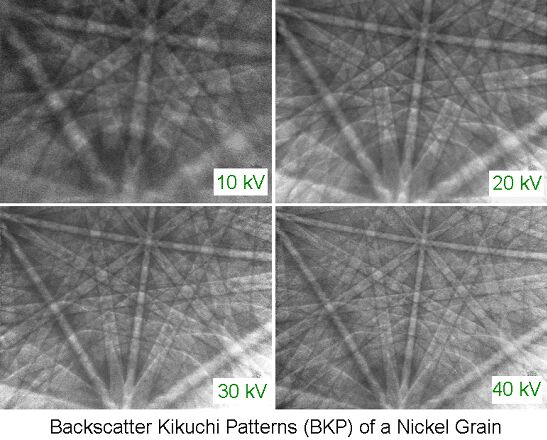The band profiles and hence the shape of the "butterfly" peaks depend on • the position of the particular band in the Kikuchi pattern, • the type of pattern, • the diffraction geometry, • the accelerating voltage, • the crystal lattice and the particular hkl The conclusion is: • One mask is not sufficient, several masks are required to analyze one pattern. • Determination of band positions and band widths is less accurate when using (inadequate) mask(s). • The wider the bands are, the larger the masks should be. Large masks, however, unduly increase computation time. => Cross-correlation with a filter mask tends to be ineffective.

2c. Conclusion

The Hough formalism obscures the simplicity of the underlying transformation. The designation of Hough transform, however, is prevalent in the EBSD literature, perhaps of not being aware of the history. The advantages of the Radon transformation include its conceptual simplicity and robustness. S is the path used for integration along the projection lines. For curves that are not straight lines, the natural extension of the Radon transformation is to project along the given curves, e.g., along hyperbolas, which are in fact the exact boundary lines of Kikuchi bands.

The general Radon transformation in Rn is an integral transformation that transforms a function f by integration along lines into a function Rf defined on the Rm. The Radon transformation has found a wide range of applications in digital image and signal processing . Examples are electron microscopy of macromolecular assemblies such as viruses and protein complexes, barcode scanners, reflection seismology, and solving hyperbolic partial differential equations. The ODF has been constructed from pole figures by Radon transformation , and the algorithm is implemented in the MTEX software package which is available for free . A popular application is the inverse Radon transformation in (axial) medical computer tomography (CT) to recover the two-dimensional image from the measured X-ray projections .

3. A simple model of Radon peak profiles illustrates band detection as a constraint task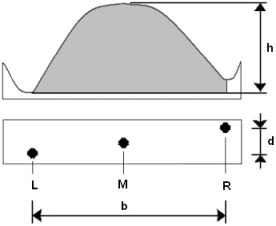The simplified peak profile model leads to a task with three constraints: The cusps L and R are local minima, and M is a local maximum:h ≥ hmin  (peak intensity is above the mean level of intensity.)d ≤ dmax (skew of the central peak, depends on hkl). bmin <≤ b <≤ bmax (range of Bragg angles φhkl, voltage U, specimen-to-screen distance L, binning) The angular distance between neighboring peaks depends on crystal lattice and range of hkl: Δρ ≤ ρmin  and  ΔΦ ≤ Φmin A simple analysis of Radon peak shapes by measuring the height h, breadth b and displacement d of the cusps L, R and central peak M. The constraints are implemented as a linked list.

4. Peak verification by Artificial Neural Networks  (ANN)

Not all peaks in the Radon space that satisfy the constraints correspond to real bands in a diffraction pattern, since the underlying peak-shape model for band profile analysis is, in the interest of short execution time, as simple as possible and there are artifacts in Radon transforms. To increase the reliability of band extraction, a layered ANN was used to verify the detected bands . ANN are superior to "sharp" verification methods based on numerical comparison of the shapes of actual and theoretical peaks, e.g., by checking a threshold value for the mean square deviation or by a cross-correlation test with a filter mask. The response function of a neuron is "soft" and "fuzzy". It can process a continuous range of intermediate values due to an adjustable sigmoid function. ANN can automatically "learn" complex relationships between data through training. They can be easily adjusted to many different peak profiles that are already present in one pattern, but in particular may vary significantly in general, especially with a change in material or phase, accelerating voltage, or diffraction set-up in general.

A set of three-layer feed-forward neural networks with back-propagation (3L FFwBP) was implemented :
• The number of input neurons, #in, (= pixels on a band profile line) is automatically increased with band width.
(The networks range from 10x6x2 to 20x11x2 neurons. The number of neurons in the hidden layer is (#in + #out)/2, and the number of output neurons, #out, is two: yes/no.)

• The neural networks were trained by back-propagation on the peak intensity distributions in Radon space of 80 real Kikuchi bands.

• The hit rate exceeds 99.2% of correctly detected bands with 9 bands per pattern.
_________

 J. Radon: Über die Bestimmung von Funktionen durch ihre Integralwerte längs gewisser Mannigfaltigkeiten. Ber. Verh. Sächs. Akad. Wiss. Leipzig, Math.-Naturw. Klasse 69 (1917) 262-267
English translation by R. Lohner: On the determination of functions from their integrals along certain manifolds. in : S.R. Deans: The Radon transform and some of its applications, Appendix A, p. 204-217. John Wiley & Sons. New York (1983).

English translation by P.C. Parks: On the determination of functions from their integral values along certain manifolds. IEEE Trans. Medical Imaging MI-5 (1986) 170-176
 R.A. Schwarzer and J. Sukkau: Automated evaluation of Kikuchi patterns by means of Radon and Fast Fourier Transformations, and verification by an artificial neural network.
Download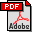N. Krieger Lassen: Automated Determination of Crystal Orientations from Electron Backscattering Patterns. PhD Thesis, Technical University of Denmark at Lyngby, 1994